#### The Chen-Fliess-Sussmann equation

There are now two expressions for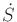, which are given by (15.128) and (15.130). By equating them,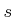equations of the form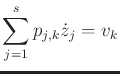(15.131)

are obtained, in which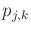is a polynomial in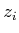variables. This makes use of the series representation for each exponential; see Example 15.23.

The evolution of the backward P. Hall coordinates is therefore given by the Chen-Fliess-Sussmann (CFS) equation: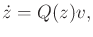(15.132)

in which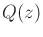is an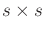matrix, and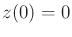. The entries inare polynomials; hence, it is possible to integrate the system analytically to obtain expressions for the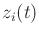.

A simple example is given, which was worked out in :

Example 15..23 (The CFS Equation for the Nonholonomic Integrator)   The extended system for the nonholonomic integrator was given in (15.115). The differential equation (15.128) for the Lie group is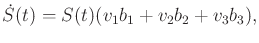(15.133)

because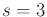.

There are two expressions for its solution. The Chen-Fliess series (15.129) becomes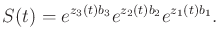(15.134)

The initial condition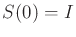is satisfied if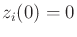forfromto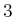. The second expression for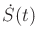is (15.130), which in the case of the nonholonomic integrator becomes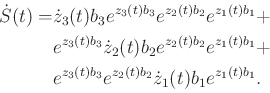(15.135)

Note that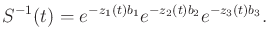(15.136)

Equating (15.133) and (15.135) yields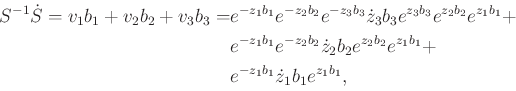(15.137)

in which the time dependencies have been suppressed to shorten the expression. The formal Lie series expansions, appropriately for the exponentials, are now used. For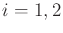,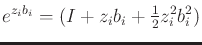(15.138)

and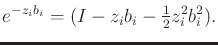(15.139)

Also,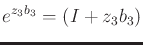(15.140)

and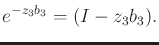(15.141)

The truncation is clearly visible in (15.140) and (15.141). The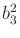terms are absent because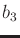is a polynomial of degree two, and its square would be of degree four.

Substitution into (15.137), performing noncommutative multiplication, and applying the Lie bracket definition yields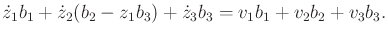(15.142)

Equating like terms yields the Chen-Fliess-Sussmann equation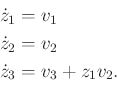(15.143)

Recall that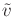is given. By integrating (15.143) from, the backward P. Hall coordinate trajectory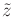is obtained.Steven M LaValle 2020-08-14